# 二维玻尔兹曼方程的不可压缩极限Incompressible Limit of the Two Dimensional Boltzmann Equation

DOI: 10.12677/PM.2020.102019, PDF, HTML, XML, 下载: 177  浏览: 308

Abstract: In this paper, we study incompressible Navier-Stokes-Fourier limit of the two dimensional Boltzmann equation. The solution of the Boltzmann equation has no high order regularity in the bounded region, so we use a recent quantitative L2-L approach with a new L4 estimate for the hydrodynamic part, to obtain uniform upper estimation of the linear part of remainder equation, and then obtain the existence of the solution of remainder equation through iteration. Finally, we get existence of the solution of the Boltzmann equation and the convergence limit.

1. 引言

${\epsilon }^{-1}\stackrel{¯}{v}\cdot {\nabla }_{x}F+\epsilon \Phi \cdot {\nabla }_{v}F={\epsilon }^{-2}Q\left(F,F\right)$, $\left(x,v\right)\in \Omega ×{ℝ}^{3}$,

${F\left(x,v\right)|}_{n\left(x\right)\cdot \stackrel{¯}{v}<0}=\sqrt{\text{2}\pi }\mu {\int }_{n\left(x\right)\cdot \stackrel{¯}{u}>0}F\left(x,u\right)\left\{n\left(x\right)\cdot \stackrel{¯}{u}\right\}\text{d}u$, $x\in \partial \Omega$, (1)

$Q\left(F,H\right)\left(v\right)={\int }_{{ℝ}^{3}}{\int }_{{\mathbb{S}}^{2}}B\left(v-u,\omega \right)\left[F\left({v}^{\prime }\right)H\left({u}^{\prime }\right)-F\left(v\right)H\left(u\right)\right]\text{d}\omega \text{d}u={Q}_{+}\left(F,H\right)\left(v\right)-{Q}_{-}\left(F,H\right)\left(v\right)$.

$\stackrel{¯}{v}\in {ℝ}^{2},\stackrel{^}{v}\in ℝ$。定义 $\mu =\frac{1}{{\left(2\pi \right)}^{\frac{3}{2}}}{\text{e}}^{-\frac{|v{|}^{2}}{2}}$$B\left(V,\omega \right)=|V\cdot \omega |$$\Phi \left(x\right)=\left({\Phi }_{1},{\Phi }_{2},0\right)$ 表示给定的外力，F是稀薄

$\stackrel{¯}{v}\cdot {\nabla }_{x}f+{\epsilon }^{2}\frac{1}{\sqrt{\mu }}\Phi \cdot {\nabla }_{v}\left[\sqrt{\mu }f\right]+{\epsilon }^{-1}Lf=\Gamma \left(f,f\right)+\epsilon \Phi \cdot v\sqrt{\mu }$，在 $\Omega$ 上，

$f={P}_{\gamma }f$，在 ${\gamma }_{-}$ 上，

${‖f‖}_{2}+{‖Pf‖}_{4}+{\epsilon }^{-1}{‖\left(I-P\right)f‖}_{\nu }+{\epsilon }^{-1/2}{|\left(1-{P}_{\gamma }\right)f|}_{2,+}+{\epsilon }^{1/2}{‖wf‖}_{\infty }\ll 1$,

$\stackrel{¯}{u}\cdot {\nabla }_{x}u+{\nabla }_{x}p=\sigma \Delta u+\Phi$${\nabla }_{x}\cdot u=0$，在 $\Omega$ 上，

$\stackrel{¯}{u}\cdot {\nabla }_{x}\theta =\kappa \Delta \theta$，在 $\Omega$ 上，

$u\left(x\right)=0,\theta \left(x\right)=0$，在 $\partial \Omega$ 上，其中 $\stackrel{¯}{u}=\left({u}_{1},{u}_{2}\right)$

2. 预备知识和主要引理

$X\underset{˜}{<}Y$ 等价于 $|X|\underset{˜}{<}CY$，C是与 $X,Y$ 无关的常数；定义 $Pf=a\sqrt{\mu }+v\cdot b\sqrt{\mu }+c\frac{{|v|}^{2}-3}{2}\sqrt{\mu }$

${\gamma }_{+}=\left\{\left(x,v\right)\in \partial \Omega ×{ℝ}^{3}:n\left(x\right)\cdot v>0\right\}$,

${\gamma }_{-}=\left\{\left(x,v\right)\in \partial \Omega ×{ℝ}^{3}:n\left(x\right)\cdot v<0\right\}$,

${\gamma }_{\text{0}}=\left\{\left(x,v\right)\in \partial \Omega ×{ℝ}^{3}:n\left(x\right)\cdot v=0\right\}$.

$\left({t}_{k+1},{x}_{k+1},{\stackrel{¯}{v}}_{k+1}\right)=\left({t}_{k}-{t}_{b}\left({x}_{k},{v}_{k}\right),{x}_{b}\left({x}_{k},{\stackrel{¯}{v}}_{k}\right),{\stackrel{¯}{v}}_{k+1}\right)$,

${X}_{cl}\left(s;t,x,\stackrel{¯}{v}\right)=\underset{k}{\sum }{1}_{\left[{t}_{k+1},{t}_{k}\right)}\left(s\right)\left\{{x}_{k}+\left(s-{t}_{k}\right){\stackrel{¯}{v}}_{k}\right\}$,

${\iint }_{\Omega ×{ℝ}^{3}}g\left(x,v\right)\sqrt{\mu }\text{d}x\text{d}v=0$, (2)

$\left[\lambda +\left(1-\tau \right){\epsilon }^{-1}\nu -\frac{1}{2}{\epsilon }^{2}\Phi \cdot v\right]f+\stackrel{¯}{v}\cdot {\nabla }_{x}f+{\epsilon }^{2}\Phi \cdot {\nabla }_{v}f+{\epsilon }^{-1}\tau Lf=g$，在 $\Omega ×{ℝ}^{\text{3}}$ 上，(3)

${f}_{-}={P}_{\gamma }f$，在 ${\gamma }_{-}$ 上，

${‖P\stackrel{\circ }{f}‖}_{\text{2}}\underset{˜}{<}{\epsilon }^{-1}{‖\left(I-P\right)f‖}_{\nu }^{}+{|\left(1-{P}_{\gamma }\right)f|}_{2,+}^{}+{‖\frac{g}{\sqrt{\nu }}‖}_{2}^{}+o\left(1\right)|〈f〉|$,(4)

$\lambda |〈f〉|\underset{\sim }{<}\left(1-\tau \right){\epsilon }^{-1}{‖f‖}_{2}$(5)

${‖P\stackrel{\circ }{f}‖}_{4}\underset{˜}{<}{\epsilon }^{-1}{‖\left(I-P\right)f‖}_{\nu }+{|\left(1-{P}_{\gamma }\right)f|}_{2,+}+{‖\frac{g}{\sqrt{\nu }}‖}_{2}+o\left(1\right)\left(|〈f〉|+{\epsilon }^{\frac{1}{2}}{‖wf‖}_{\infty }\right)$, (6)

${\stackrel{¯}{\omega }}_{\tau }=\lambda +\left(1-\tau \right){\epsilon }^{-1}\nu -\frac{1}{2}{\epsilon }^{2}\Phi \cdot v$，根据格林公式(见参考文献 )，得

${\iint }_{\Omega ×{ℝ}^{3}}\left\{\stackrel{¯}{v}\cdot {\nabla }_{x}f+{\epsilon }^{2}\Phi \cdot {\nabla }_{v}f\right\}\psi +\left\{\stackrel{¯}{v}\cdot {\nabla }_{x}\psi +{\epsilon }^{2}\Phi \cdot {\nabla }_{v}\psi \right\}f={\int }_{{\gamma }^{+}}f\psi -{\int }_{{\gamma }^{-}}f\psi$.

$\begin{array}{l}{\iint }_{\Omega ×{ℝ}^{3}}{\stackrel{¯}{\omega }}_{\tau }f\psi -\stackrel{¯}{v}\cdot f{\nabla }_{x}\psi -{\epsilon }^{2}f\Phi \cdot {\nabla }_{v}\psi +{\int }_{{\gamma }^{+}}f\psi -{\int }_{{\gamma }^{-}}f\psi \\ =-{\epsilon }^{-1}\tau {\iint }_{\Omega ×{ℝ}^{3}}\psi L\left(I-P\right)f+{\iint }_{\Omega ×{ℝ}^{3}}\psi g\end{array}$. (7)

$a-〈f〉=\stackrel{\circ }{a}$，则 $P\stackrel{\circ }{f}=\left(\stackrel{\circ }{a}\text{\hspace{0.17em}}+v\cdot b+c\frac{{|v|}^{2}-3}{2}\right)\sqrt{\mu }$，分以下三步证明。

${‖c‖}_{4}\underset{˜}{<}o\left(1\right){‖Pf‖}_{4}+{\epsilon }^{-1}{‖\left(I-P\right)f‖}_{\nu }+{‖\left(1-P\right)f‖}_{4}+{|\left(1-{P}_{\gamma }\right)f|}_{2,+}+{‖\frac{g}{\sqrt{\nu }}‖}_{2}$. (8)

$N=2$ 时，我们想要 ${p}^{*}=2$，所以由 $\frac{1}{p}-\frac{1}{2}\le \frac{1}{2}$，得 $p\ge 1$，这里我们取 $p=\frac{4}{3}$，则对于任意的 $q\in \left[\frac{4}{3},2\right]$

${‖\nabla {\phi }_{c,4}‖}_{q}\underset{˜}{<}{‖\nabla {\phi }_{c,4}‖}_{{W}^{2,\frac{4}{3}}}$,

${‖\nabla {\phi }_{c,4}‖}_{\text{2}}\underset{˜}{<}{‖{\phi }_{c,4}‖}_{{W}^{2,\frac{4}{3}}}\underset{˜}{<}{‖{c}^{3}‖}_{\frac{4}{3}}={‖c‖}_{{}_{4}}^{3}$,(9)

(7)的右边 $\underset{˜}{<}{‖c‖}_{4}^{3}\left({\epsilon }^{-1}{‖\left(I-P\right)f‖}_{\nu }+{‖\frac{g}{\sqrt{\nu }}‖}_{2}\right)$(10)

$\psi ={\psi }_{c,\text{4}}=\left({|v|}^{2}-{\beta }_{c}\right)\sqrt{u}\stackrel{¯}{v}\cdot {\nabla }_{x}{\phi }_{c,4}$ 代入(7)，则(7)的左边可以写成如下形式：

${\iint }_{\partial \Omega ×{ℝ}^{3}}\left(n\left(x\right)\cdot \stackrel{¯}{v}\right)\left({|v|}^{2}-{\beta }_{c}\right)\sqrt{\mu }\underset{i=1}{\overset{2}{\sum }}{v}_{i}{\partial }_{i}{\phi }_{c,4}\left(x\right)\text{ }f\text{d}{S}_{x}\text{d}v$ (11)

$+{\int }_{\Omega ×{ℝ}^{3}}\left[\lambda +\left(1-\tau \right){\epsilon }^{-1}\nu \right]f\left({|v|}^{2}-{\beta }_{c}\right)\sqrt{\mu }\underset{i=1}{\overset{2}{\sum }}{v}_{i}\partial {\phi }_{c,4}\left(x\right)\text{ }\text{d}x\text{d}v$ (12)

$-{\iint }_{\Omega ×{ℝ}^{3}}\left({|v|}^{2}-{\beta }_{c}\right)\sqrt{\mu }\left\{\underset{i,j=1}{\overset{2}{\sum }}{v}_{i}{v}_{j}{\partial }_{ij}{\phi }_{c,4}\left(x\right)\right\}f\text{d}x\text{d}v$ (13)

$-{\epsilon }^{2}\underset{i,j}{\sum }{\iint }_{\Omega ×{ℝ}^{3}}\sqrt{\mu }{\Phi }_{i}\left\{{v}_{i}{v}_{j}\left({|v|}^{2}-{\beta }_{c}\right){\partial }_{j}{\phi }_{c,4}\left(x\right)+\left[{\delta }_{i,j}\left({|v|}^{2}-{\beta }_{c}\right)+2{v}_{i}{v}_{j}\right]{\partial }_{j}{\phi }_{c,4}\left(x\right)\right\}f$. (14)

$f=\left(a+v\cdot b+c\frac{{|v|}^{2}-3}{2}\right)\sqrt{\mu }+\left(I-P\right)f$，在 $\Omega ×{ℝ}^{3}$ 上， (15)

${f}_{\gamma }={P}_{\gamma }f+{1}_{{\gamma }^{+}}\left(1-{P}_{\gamma }\right)f$，在 $\gamma$ 上。 (16)

${\beta }_{c}=5$ 使得 ${\int }_{{ℝ}^{3}}\left({|v|}^{2}-{\beta }_{c}\right){v}_{i}^{2}u\left(v\right)\text{d}v=0,i=1,2$，则由奇函数性质，(13)表达式变成如下形式：

$\left(\text{13}\right)=-\underset{i=1}{\overset{2}{\sum }}{\int }_{{ℝ}^{3}}\left({|v|}^{2}-5\right){v}_{i}^{2}\frac{{|v|}^{2}-3}{2}\mu \left(v\right)\text{d}v{\int }_{\Omega }{\partial }_{ii}{\phi }_{c,4}\left(x\right)c\left(x\right)\text{d}x$ (17)

$-\underset{i=1}{\overset{2}{\sum }}{\iint }_{\Omega ×{ℝ}^{3}}\left({|v|}^{2}-5\right){v}_{i}\sqrt{\mu }\left(\stackrel{¯}{v}\cdot {\nabla }_{x}\right){\partial }_{i}{\phi }_{c,4}\left(I-P\right)f$. (18)

$\left(\text{17}\right)=-5{\int }_{\Omega }{\Delta }_{x}{\phi }_{c,4}c=5{‖c‖}_{4}^{4}$. (19)

$\left(\text{18}\right)\le {‖{\nabla }^{2}{\phi }_{c,2}‖}_{\frac{4}{3}}{‖\left(I-P\right)f‖}_{4}\le {‖c‖}_{4}^{3}{‖\left(I-P\right)f‖}_{4}$. (20)

${\beta }_{c}=5$ 时，由( ${v}_{i}{v}_{j}\left(i\ne j\right)$ 的奇性可得

${\iint }_{\partial \Omega ×{ℝ}^{3}}\left(n\left(x\right)\cdot \stackrel{¯}{v}\right)\left({|v|}^{2}-{\beta }_{c}\right)\sqrt{\mu }\underset{i=1}{\overset{2}{\sum }}{v}_{i}{\partial }_{i}{\phi }_{c,4}\left(x\right){P}_{\gamma }f\text{d}{S}_{x}\text{d}v=\text{0}$.

${\left({\int }_{\partial \Omega }\text{d}S\left(x\right){|u|}^{\frac{p\left(N-1\right)}{N-p}}\right)}^{\frac{N-p}{p\left(N-1\right)}}\le C\left(N,p\right){\left({\int }_{\Omega }\text{d}x{|u|}^{p}+{\int }_{\Omega }\text{d}x{|\nabla u|}^{p}\right)}^{\frac{1}{p}}$.

$p=\frac{4}{3}$$N=2$ 时， $\frac{p\left(N-1\right)}{N-p}=2$

$u=\nabla {\phi }_{c,4}$，由(9)得 ${‖{\nabla }_{x}{\phi }_{c,4}‖}_{{L}^{\frac{2}{\text{3}}}\left(\partial \Omega \right)}\underset{˜}{<}{‖{\nabla }_{x}{\phi }_{c,4}‖}_{{W}^{1,\frac{4}{3}}\left(\Omega \right)}\underset{˜}{<}{‖c‖}_{4}^{3}$，所以

$\left(\text{11}\right)\underset{˜}{<}{\mu }^{\frac{1}{2}}{|\left(1-{P}_{\gamma }\right)f|}_{2,+}{|{\nabla }_{x}{\phi }_{c,4}|}_{2,+}\underset{˜}{<}{|\left(1-{P}_{\gamma }\right)f|}_{2,+}{‖c‖}_{4}^{3}$. (21)

$\left(\text{12}\right)\underset{˜}{<}\left(\lambda +o\left(1\right)\right)×\left\{{‖Pf‖}_{4}+{‖\left(I-P\right)f‖}_{4}\right\}{‖c‖}_{4}^{3}$, (22)

$\left(\text{14}\right)\le {\epsilon }^{2}\left[{‖c‖}_{4}^{4}+{‖c‖}_{4}^{3}{‖\left(I-P\right)f‖}_{4}\right]{‖\Phi ‖}_{\infty }$. (23)

${‖c‖}_{4}\underset{˜}{<}o\left(1\right){‖Pf‖}_{4}+{|\left(I-{P}_{\gamma }\right)f|}_{2,+}+{\epsilon }^{-1}{‖\left(I-P\right)f‖}_{\nu }+{‖\left(I-P\right)f‖}_{4}+{‖\frac{g}{\sqrt{\nu }}‖}_{2}$.

${‖b‖}_{4}\underset{˜}{<}o\left(1\right){‖Pf‖}_{4}+{|\left(I-{P}_{\gamma }\right)f|}_{2,+}+{\epsilon }^{-1}{‖\left(I-P\right)f‖}_{\nu }+{‖\left(I-P\right)f‖}_{4}+{‖\frac{g}{\sqrt{\nu }}‖}_{2}$. (24)

$\psi ={\psi }_{b,\text{4}}^{i,j}\equiv \left({v}_{i}^{2}-{\beta }_{b}\right)\sqrt{\mu }{\partial }_{j}{\phi }_{b,\text{4}}^{j},\text{\hspace{0.17em}}i,j=1,2$, (25)

(7)右边 $\le {‖b‖}_{4}^{3}\left({\epsilon }^{-1}{‖\left(I-P\right)‖}_{\nu }+{‖\frac{g}{\sqrt{\nu }}‖}_{2}\right)$(26)

${\iint }_{\partial \Omega ×{ℝ}^{\text{3}}}\left(n\left(x\right)\cdot \stackrel{¯}{v}\right)\left({v}_{i}^{2}-{\beta }_{b}\right)\sqrt{\mu }{\partial }_{j}{\phi }_{b,4}^{j}\left[\left(1-{P}_{\gamma }\right)f\right]{1}_{{\gamma }_{+}}$ (27)

$+{\iint }_{\Omega ×{ℝ}^{\text{3}}}\left[\lambda +\left(1-\tau \right){\epsilon }^{-1}v\right]\text{ }f\left({v}_{i}^{2}-{\beta }_{b}\right)\sqrt{\mu }{\partial }_{j}{\phi }_{b,4}^{j}$ (28)

$+{\iint }_{\Omega ×{ℝ}^{\text{3}}}\left({v}_{i}^{2}-{\beta }_{b}\right)\sqrt{\mu }\left\{\underset{l}{\sum }{v}_{l}{\partial }_{lj}{\phi }_{b,4}^{j}\right\}f$ (29)

$-{\iint }_{\Omega ×{ℝ}^{3}}{\epsilon }^{2}\sqrt{\mu }f\left[\Phi \cdot v\left({v}_{i}^{2}-{\beta }_{b}\right){\partial }_{j}{\phi }_{b,4}^{j}+2{\Phi }_{i}{v}_{i}\right]{\partial }_{j}{\phi }_{b,4}^{j}$ (30)

$\left(\text{27}\right)\underset{˜}{<}{|\left(I-{P}_{\gamma }\right)f|}_{2,+}{|{\nabla }_{x}{\phi }_{b,4}|}_{2,+}\le {|\left(I-{P}_{\gamma }\right)f|}_{2,+}{‖b‖}_{4}^{3}$. (31)

$\left(\text{28}\right)\underset{˜}{<}\left(\lambda +o\left(1\right)\right)\left({‖Pf‖}_{4}+{‖\left(I-P\right)f‖}_{4}\right){‖b‖}_{4}^{3}$. (32)

$\left(\text{29}\right)=-\underset{i=1,2}{\sum }\left[{\iint }_{\Omega ×{ℝ}^{\text{3}}}\left({v}_{i}^{2}-{\beta }_{b}\right){v}_{l}^{2}\mu {\partial }_{lj}{\phi }_{b,4}^{j}\left(x\right){b}_{l}+{\iint }_{\Omega ×{ℝ}^{\text{3}}}\left({v}_{i}^{2}-{\beta }_{b}\right){v}_{l}\sqrt{\mu }{\partial }_{lj}{\phi }_{b,4}^{j}\left(x\right)\left(I-P\right)f\right]$. (33)

(33)中第一项 $=2{\int }_{\Omega }\left({\partial }_{i}{\partial }_{j}{\Delta }^{-1}{b}_{j}^{3}\left(x\right)\right){b}_{i}$(34)

(33)中第二项 $\underset{˜}{<}{‖{\nabla }^{2}{\phi }_{b,4}‖}_{\frac{4}{3}}\cdot {‖\left(I-P\right)f‖}_{4}\le {‖b‖}_{4}^{3}\cdot {‖\left(I-P\right)f‖}_{4}$(35)

$\left(\text{3}0\right)\underset{˜}{<}{\epsilon }^{2}{‖\Phi ‖}_{\infty }\left({‖b‖}_{4}^{4}+{‖b‖}_{4}^{3}\cdot {‖\left(I-P\right)f‖}_{4}^{4}\right)$. (36)

$|{\int }_{\Omega }\left({\partial }_{i}{\partial }_{j}{\Delta }^{-1}{b}_{j}^{3}\right){b}_{i}|\underset{˜}{<}{‖b‖}_{4}^{3}\left({\epsilon }^{-1}{‖\left(I-P\right)f‖}_{\nu }+{‖\frac{g}{\sqrt{\nu }}‖}_{2}+{|\left(I-{P}_{\gamma }\right)f|}_{2,+}+o\left(1\right){‖Pf‖}_{4}\right)$. (37)

(7)右边 $\underset{˜}{<}{‖b‖}_{4}^{3}\left({\epsilon }^{-1}{‖\left(I-P\right)f‖}_{\nu }+{‖\frac{g}{\sqrt{\nu }}‖}_{2}\right)$

$\begin{array}{c}-{\iint }_{\Omega ×{ℝ}^{3}}\stackrel{¯}{v}\cdot {\nabla }_{x}\psi f=-{\iint }_{\Omega ×{ℝ}^{3}}{|v|}^{2}{v}_{i}{v}_{j}\sqrt{\mu }\left\{\underset{l=1}{\overset{2}{\sum }}{v}_{l}{\partial }_{lj}{\phi }_{b,4}^{i}\right\}f\\ =-{\iint }_{\Omega ×{ℝ}^{3}}{|v|}^{2}{v}_{i}^{2}{v}_{j}^{2}\mu \left[{\partial }_{ij}{\phi }_{b,4}^{i}{b}_{j}+{\partial }_{jj}{\phi }_{b,4}^{i}{b}_{j}\right]\\ \text{\hspace{0.17em}}\text{\hspace{0.17em}}\text{ }-\underset{l=1}{\overset{2}{\sum }}{\iint }_{\Omega ×{ℝ}^{3}}{|v|}^{2}{v}_{i}{v}_{j}{v}_{l}\sqrt{\mu }{\partial }_{lj}{\phi }_{b,4}^{i}\left(x\right)\left(I-P\right)f\end{array}$. (38)

(38)中第一项 $=-4\left[{\int }_{\Omega }\left({\partial }_{i}{\partial }_{j}{\Delta }^{-1}{b}_{i}^{\text{3}}\right){b}_{j}+\left({\partial }_{j}{\partial }_{j}{\Delta }^{-1}{b}_{i}^{\text{3}}\right){b}_{i}\right]$

$\begin{array}{c}{‖\stackrel{\circ }{a}‖}_{\text{4}}\underset{˜}{<}o\left(1\right){‖Pf‖}_{\text{4}}+{‖\left(I-P\right)f‖}_{\text{4}}+{\epsilon }^{-1}{‖\left(I-P\right)f‖}_{2}\\ \text{\hspace{0.17em}}\text{\hspace{0.17em}}+{|\left(I-{P}_{\gamma }\right)f|}_{2,+}^{2}+{‖\frac{g}{\sqrt{\nu }}‖}_{2}+o\left(1\right)|〈f〉|\end{array}$. (39)

(7)右边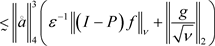.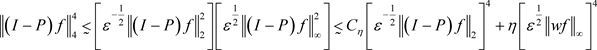,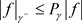,.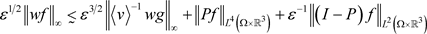. (40). (41)..,.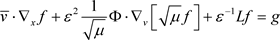，在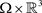上，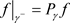,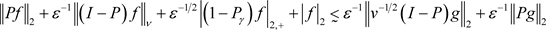, (42)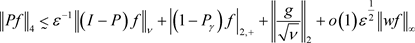. (43)

3. 定理1.1的证明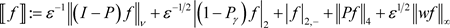.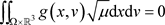,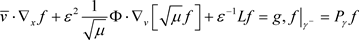,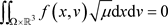,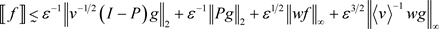.(44)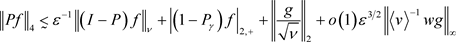. (45), (46).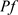关于v的强衰减性，可以得到，对于任意的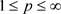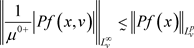，其中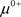表示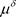。对于固定v，由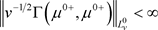，我们有.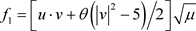的系数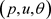满足INSF方程和边界。由于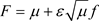，F的存在唯一性

  Guo, Y. (2010) Decay and Continuity of the Boltzmann Equation in Bounded Domains. Archive for Rational Mechanics and Analysis, 197, 713-809. https://doi.org/10.1007/s00205-009-0285-y  Bardos, C., Golse, F. and Levermore, D. (1991) Fluid Dynamic Limits of Kinetic Equations I: Formal Derivations. Journal of Statistical Physics, 63, 323-344. https://doi.org/10.1007/BF01026608  Bardos, C., Golse, F. and Levermore, D. (1993) Fluid Dynamic Limits of Kinetic Equations II: Convergence Proofs for the Boltzmann Equation. Communications on Pure and Applied Mathematics, 46, 667-753. https://doi.org/10.1002/cpa.3160460503  Golse, F. and Saint-Raymond, L. (2004) The Navier-Stokes Limit of the Boltzmann Equation for Bounded Collision Kernels. Inventiones Mathematicae, 155, 81-161. https://doi.org/10.1007/s00222-003-0316-5  Golse, F. (2005) Hydrodynamic Limits. European Congress of Mathematics. Eur. Math. Soc, 699-717. https://doi.org/10.4171/009-1/44  Esposito, R., Guo, Y., Kim, C. and Marra, R. (2018) Stationary Solutions to the Boltzmann Equation in the Hydrodynamic Limit. Annals of PDE, 4, 1-55. https://doi.org/10.1007/s40818-017-0037-5  Leoni, G. (2009) A First Course in Sobolev Spaces. AMS Graduate Studies in Mathematics, 105. https://doi.org/10.1090/gsm/105  Krylov, N.V. (2008) Lectures on Elliptic and Parabolic Equations in Sobolev Spaces. AMS Graduate Studies in Mathematics, 96. https://doi.org/10.1090/gsm/096